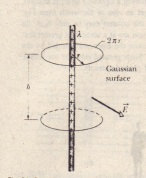# Applying Gauss’ Law: Cylindrical Symmetry

Applying Gauss’ Law:
Cylindrical Symmetry

section of an infinitely long cylindrical plastic rod with a uniform positive linear charge density A. Let us find an expression for the magnitude of the electric field E at a distance r from the axis of the rod Our Gaussian surface should match the symmetry of the problem which is cylindrical. We choose a circular cylinder of radius r and length 17 coaxial with the rod The Gaussian surface must be closed. so we include two end caps as part of
the surface Imagine now that while you are not watching, someone rotates the plastic rod around its longitudinal axis or turns it end for end When you look again at the rod you will not be able to detect any change We conclude from this symmetry that the only uniquely specified direction in this problem is along a radial line Thus at every point on the cylindrical part of the Gaussian surface. E must have the same magnitude E and (for a positively charged rod) must be directed radially outward Since Trotter is the cylinder’s circumference and II is its height, the area A of the cylindrical surface is The flux of E through this cylindrical surface is then
<1) = EA cos () = E(27Tl’h) cos 0 = E(27Tl·h) There is no flux through the end caps because E being radially directed is parallel to the end caps at every point The charge enclosed by the surface is Xh so Gauss’ lawThis is the electric field due to an infinitely long straight line of charge at a point that is a radial distance r from the line. The direction of E is radially outward from the line of charge if the charge is positive and radially inward if it is negative also approximates the field of a fill ire line of charge at   re not too near the ends (compared with the distance from the line).Gaussian surface in the form of a closed cylinder surrounds a section of a very long uniformly charge cylindrical plastic rod.